# Conceptual Graph Examples

Conceptual graphs are formally defined in an abstract syntax that is independent of any notation, but the formalism can be represented in several different concrete notations. This document illustrates CGs by means of examples represented in the graphical display form (DF), the formally defined conceptual graph interchange form (CGIF), and the compact, but readable linear form (LF). Every CG is represented in each of these three forms and is translated to a logically equivalent representation in predicate calculus and in the Knowledge Interchange Format (KIF). For the formal definition of conceptual graphs and the various notations for representing them, see the draft proposed American National Standard. For examples of an English-like notation for representing logic, see the web page on controlled English.

## List of Examples

Following are some sample sentences that are represented in each of the notations: CGs, KIF, and predicate calculus. Click on the sentence to go directly to its representation.

## 1. A cat is on a mat.

In the display form (DF), concepts are represented by rectangles: the concept [Cat] represents a instance of a cat, and [Mat] represents an instance of a mat. Conceptual relations are represented by circles or ovals: the conceptual relation (On) relates a cat to a mat. The arcs that link the relations to the concepts are represented by arrows: the first arc has an arrow pointing toward the relation, and the second arc has an arrow pointing away from the relation. If a relation has more than two arcs, the arcs are numbered.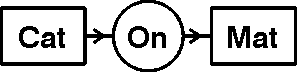In the linear form (LF), concepts are represented by square brackets instead of boxes, and the conceptual relations are represented by parentheses instead of circles:

```[Cat]Û(On)Û[Mat].
```

Both DF and LF are designed for communication with humans or between humans and machines. For communication between machines, the conceptual graph interchange form (CGIF) has a syntax that uses coreference labels to represent the arcs:

```[Cat: *x] [Mat: *y] (On ?x ?y)
```

The symbols *x and *y are called defining labels. The matching symbols ?x and ?y are the bound labels that indicate references to the same instance of a cat x or a mat y. To reduce the number of coreference labels, CGIF also permits concepts to be nested inside the relation nodes:

```(On [Cat] [Mat])
```

The display form in Figure 1 represents the abstract CG most directly. All the variations of LF and CGIF represent stylistically different, but logically equivalent ways of linearizing the same abstract graph. All these variations are accommodated by the LF grammar and the CGIF grammar, which are defined in the CG standard.

CGIF is intended for transfer between computer systems that use CGs as their internal representation. For communication with systems that use other internal representations, CGIF can be translated to another logic-based formalism called the Knowledge Interchange Format (KIF):

```(exists ((?x Cat) (?y Mat)) (On ?x ?y))
```

Although DF, LF, CGIF, and KIF look very different, their semantics is defined by the same logical foundations. They can all be translated to a statement of the following form in typed predicate calculus:

```(\$x:Cat)(\$y:Mat)on(x,y).
```
Any statement expressed in any one of these notations can be automatically translated to a logically equivalent statement in any of the others. Formatting and stylistic information, however, may be lost in translations between DF, LF, CGIF, KIF, and predicate calculus.

## 2. Every cat is on a mat.

The default quantifier in a concept is the existential \$, which is normally represented by a blank. The concept [Cat] without anything in the referent field is logically equivalent to the concept [Cat: \$], which asserts the proposition that there exists a cat. Other quantifiers, such as the universal ", are called defined quantifiers because they can be defined in terms of conceptual graphs containing only the default existential. In Figure 2, the concept [Cat: "] represents the phrase every cat, and the complete CG represents the sentence Every cat is on a mat.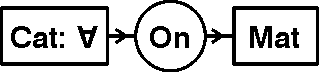In the linear form (LF), the universal quantifier may be represented by the symbol " if it is available. Otherwise, it is represented by the symbol @every. Both of the following CGs are semantically identical:

```[Cat: "]Û(On)Û[Mat].

[Cat: @every]Û(On)Û[Mat].
```

Since CGIF is expressible in the 7-bit subset of ASCII or Unicode, the character " must be represented by @every in CGIF:

```[Cat: @every*x] [Mat: *y] (On ?x ?y)
```

As in Figure 1, CGIF permits concepts to be nested inside the relation nodes:

```(On [Cat: @every] [Mat])
```

In all these examples, the universal quantifier @every or " includes the default existential quantifiers in the same context within its scope. The scope is enforced by the definition of the quantifier @every in terms of the existential. When the definition is expanded, the CG in Figure 2 is expanded to a CG that can be represented by the following LF graph:

```~[ [Cat: *x]
~[ [?x]Û(On)Û[Mat] ]].
```
Literally, this CG may be read It is false that there exists a cat x that is not on a mat. An alternate reading treats the two nested negations as an implication: If there exists a cat x, then x is on a mat. Following is a CGIF representation of the expanded CG:
```~[ [Cat: *x]  ~[ (On ?x [Mat]) ]].
```
All of these forms are logically equivalent to the original CG in Figure 2.

Since KIF has a universal quantifier, it is not necessary to expand the defined quantifier @every before translating a CG to KIF. Following is the KIF translation of Figure 2:

```(forall ((?x Cat)) (exists ((?y Mat)) (On ?x ?y)))
```
This KIF statement is logically equivalent to the KIF statement that results from translating the expanded CG:
```(not (exists ((?x Cat)) (not (exists ((?y Mat)) (On ?x ?y)))))
```

The original CG in Figure 2 may be represented by the following formula in typed predicate calculus:

```("x:Cat)(\$y:Mat)on(x,y).
```
This formula is logically equivalent to the formula that represents the expanded CG:
```~(\$x:Cat)~(\$y:Mat)on(x,y).
```

## 3. John is going to Boston by bus.

Figure 3 shows a conceptual graph with four concepts: [Go], [Person: John], [City: Boston], and [Bus]. It has three concdeptual relations: (Agnt) relates [Go] to the agent John, (Dest) relates [Go] to the destination Boston, and (Inst) relates [Go] to the instrument bus.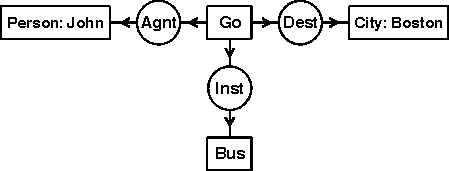Since the concept [Go] is attached to three conceptual relations, the linear form cannot be drawn in a straight line, as in Figure 1. Instead, a hyphen at the end of the first line indicates that the relations attached to [Go] are continued on subsequent lines.

```[Go]-
(Agnt)Û[Person: John]
(Dest)Û[City: Boston]
(Inst)Û[Bus].
```

This example resembles frame notation, but LF also permits coreference labels to represent the cross references needed to represent arbitrary graphs.

In the following CGIF representation for Figure 3, each concept has its own defining label:

```[Go: *x] [Person: John *y] [City: Boston *z] [Bus: *w]
(Agnt ?x ?y) (Dest ?x ?z) (Inst ?x ?z)
```

By nesting some of the concepts inside the relations, the CGIF form can be limited to just a single defining label *x and a bound label ?x inside each relation node:

```[Go *x] (Agnt ?x [Person: John]) (Dest ?x [City: Boston]) (Inst ?x [Bus])
```

The display form in Figure 3 represents the abstract CG most directly. All the variations of LF and CGIF represent different, but logically equivalent ways of linearizing the same abstract graph.

The version of CGIF that assigns a separate defining label to each concept usually has the most direct mapping to KIF:

```(exists ((?x Go) (?y Person) (?z City) (?w Bus))
(and (Name ?y John) (Name ?z Boston)
(Agnt ?x ?y) (Dest ?x ?z) (Inst ?x ?w)))
```

Following is the corresponding formula in typed predicate calculus:

```(\$x:Go)(\$y:Person)(\$z:City)(\$w:Bus)
(name(y,'John') ì name(z,'Boston') ì
agnt(x,y) ì dest(x,z) ì inst(x,w))
```
For a list of the relations that connect the concepts corresponding to verbs to the concepts of their participants, see the web page on thematic roles.

## 4. A person is between a rock and a hard place.

The between relation (Betw) is a triadic relation, whose first two arcs are linked to concepts of entities that occur on either side of the entity represented by the concept linked to the third arc. For a conceptual relation with n arcs, the first n-1 arcs have arrows that point toward the circle, and the n-th or last arc points away.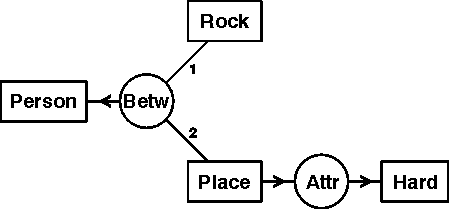In LF, Figure 4 may be represented in the following form:

```[Person]˜(Betw)-
˜1-[Rock]
˜2-[Place]Û(Attr)Û[Hard].
```
The hyphen after the relation indicates that its other arcs are continued on subsequent lines. The two arcs that point towards the relation are numbered 1 and 2. The arc that points away is the last or third arc; the number 3 may be omitted, since it is implied by the outward pointing arrow. For monadic relations, both the number 1 and the arrow pointing towards the circle are optional. For dyadic relations, the arcs are either numbered 1 and 2, or the first arc points towards the circle and the second arc points away.

CGIF allows any number of concepts to be nested inside the relations:

```(Betw [Rock] [Place *x] [Person])  (Attr ?x [Hard])
```
For relations with more than 2 arcs, CGIF notation is more compact than the multiline LF notation. Therefore, most LF implementations allow CGIF notation to be mixed with the arrow notation:
```[Place: *x]Û(Attr)Û[Hard]  (Betw [Rock] ?x [Person]).
```
In the CG standard, the only notation that must be standardized is CGIF, since every implementation must recognize exactly the same forms. The LF and DF notations are specified only in an informative annex to the CG standard. Therefore, implementers may experiment with different variations in an attempt to improve readability or convenience. Nevertheless, too much variation may make it harder for human readers to switch from one version to another.

Following is the KIF representation:

```(exists ((?x person) (?y rock) (?z place) (?w hard))
(and (betw ?y ?z ?x) (attr ?z ?w)))
```
And following is the corresponding formula in predicate calculus:
```(\$x:Person)(\$y:Rock)(\$z:Place)(\$w:Hard)
(betw(y,z,x) ì attr(z,w))
```
To emphasize the correspondence between CGs and KIF, it is possible to write CGIF in a form in which the concept nodes with their quantifiers and coreference labels precede the relation nodes:
```[Person *x] [Rock *y] [Place *z] [Hard *w]
(betw ?y ?z ?x) (attr ?z ?w)
```

## 5. Tom believes that Mary wants to marry a sailor.

A context is a concept with a nested conceptual graph that describes the referent. In Figure 4, the concept of type Proposition is a context that describes a proposition that Tom believes. Inside that context is another context of type Situation, which describes a situation that Tom believes Mary wants. The resulting CG represents the sentence Tom believes that Mary wants to marry a sailor.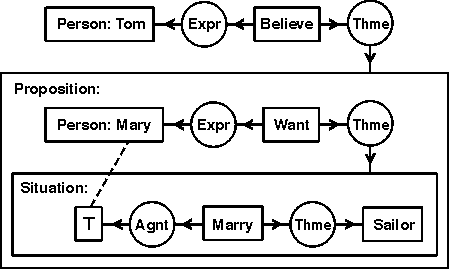In Figure 5, Tom is the experiencer (Expr) of the concept [Believe], which is linked by the theme relation (Thme) to a proposition that Tom believes. The proposition box contains another conceptual graph, which says that Mary is the experiencer of [Want], which has as theme a situation that Mary hopes will come to pass. That situation is described by another nested graph, which says that Mary (represented by the concept [T]) marries a sailor. The dotted line, called a coreference link, shows that the concept [T] in the situation box refers to the same individual as the concept [Person: Mary] in the proposition box. Following is the corresponding linear form:

```[Person: Tom]˜(Expr)˜[Believe]Û(Thme)-
[Proposition:  [Person: Mary *x]˜(Expr)˜[Want]Û(Thme)-
[Situation:  [?x]˜(Agnt)˜[Marry]Û(Thme)Û[Sailor] ]].
```
Both the display form and the linear form follow the same rules for the scope of quantifiers. The part of the graph outside the nested contexts contains three concepts: [Person: Tom], [Believe], and the proposition that Tom believes. That part of the graph asserts information that is assumed to be true of the real world.

Inside the proposition box are three more concepts: [Person: Mary], [Want], and the situation that Mary wants. Since those three are only asserted within the context of Tom's belief, the graph does not imply that they must exist in the real world. Since Mary is a named individual, one might give her the benefit of the doubt and assume that she also exists; but her desire and the situation she supposedly desires exist in the context of Tom's belief. If his belief is false, the referents of those concepts might not exist in the real world. Inside the context of the desired situation are the concepts [Marry] and [Sailor], whose referents exist within the scope of Mary's desire, which itself exists only within the scope of Tom's belief.

Following is the CGIF representation for Figure 5:

```[Person: *x1 'Tom'] [Believe *x2] (Expr ?x2 ?x1)
(Thme ?x2 [Proposition:
[Person: *x3 'Mary'] [Want *x4] (Expr ?x4 ?x3)
(Thme ?x4 [Situation:
[Marry *x5] (Agnt ?x5 ?x3) (Thme ?x5 [Sailor]) ]) ])
```

Following is the KIF statement:

```(exists ((?x1 person) (?x2 believe))
(and (expr ?x2 ?x1)
(thme ?x2
(exists ((?x3 person) (?x4 want) (?x8 situation))
(and (name ?x3 'Mary) (expr ?x4 ?x3) (thme ?x4 ?x8)
(dscr ?x8 (exists ((?x5 marry) (?x6 sailor))
(and (Agnt ?x5 ?x3) (Thme ?x5 ?x6)))))))))
```
Following is the predicate calculus formula:
```(\$x1:Person)(\$x2:Believe)(expr(x1,x2) ì
thme(x2, (\$x3:Person)(\$x4:Want)(\$x8:Situation)
(name(x3,'Mary') ì expr(x4,x3) ì thme(x4,x8) ì
dscr(x8, (\$x5:Marry)(\$x6:Sailor)
(agnt(x5,x3) ì thme(x5,x6))))))
```

For further discussion of contexts and their representation in conceptual graphs and predicate calculus, see Chapter 5 of the book Knowledge Representation.

Copyright ©1999 by John F. Sowa. Anyone who is teaching or learning CGs or implementing tools that generate or interpret CGs is welcome to use these examples as illustrations or test cases. A license is hereby granted to anyone who would like to use the graphical images or the text-based representations in this document for any purpose, private or commercial, provided that the author and the URL of this document are cited in any publication that explains or accompanies such use.Last Modified: